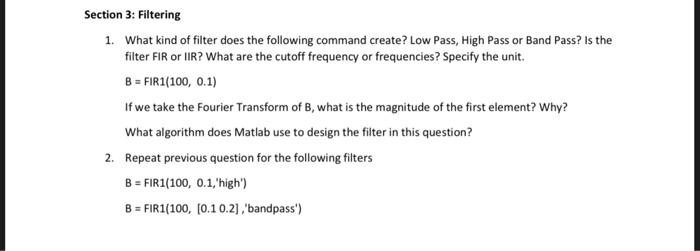Home / Expert Answers / Electrical Engineering / 1-what-kind-of-filter-does-the-following-command-create-low-pass-high-pass-or-band-pass-is-the-pa447

# (Solved): 1. What kind of filter does the following command create? Low Pass, High Pass or Band Pass? Is the ...1. What kind of filter does the following command create? Low Pass, High Pass or Band Pass? Is the filter FIR or IIR? What are the cutoff frequency or frequencies? Specify the unit. $B=\operatorname{FIR} 1(100,0.1)$ If we take the Fourier Transform of $$\mathrm{B}$$, what is the magnitude of the first element? Why? What algorithm does Matlab use to design the filter in this question? 2. Repeat previous question for the following filters $\begin{array}{l} B=F I R 1(100,0.1 \text {,'high') } \\ B=F I R 1(100,[0.10 .2] \text {,'bandpass') } \\ \end{array}$

We have an Answer from Expert

1. The command FIR1(100, 0.1) creates a low pass filter with a cut